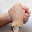# 【论文分享】图上做伪标签生成缓解推荐冷启动

## 具体解法

### 定义

• user 集合：$\mathcal{U}$
• item 集合：$\mathcal{I}$
• $u$ 可观测到的 item 集合：$\mathcal{I}^{+}_u$
• $u$ 不可观测到的 item 集合：$\mathcal{I} \backslash \mathcal{I}^{+}_u$
• 正样本集：$\mathcal{I}^{+}_u$
• 负样本集：$\mathcal{I}^{-}_u$
• 伪样本集：$\mathcal{I}^{±}_u$

### 基于伪标签的半监督学习#### 损失函数### 基于知识图谱的流行度采样

#### 伪标签采样#### 负样本采样#### mini-batch 构造策略### co-training

#### 训练过程

1. 采样两个 mini-batch $B_f, B_g$，其伪标签分别由模型 $f, g$ 预测得到。

2. 将 $B_f$ 输入 $g$ 训练，将 $B_g$ 输入 $f$ 训练。

#### 损失函数## 实验## 参考链接

### 8 只已被捕捉

•Dabenshi Chrome | 112.0.0.0 Windows 10/11

论文…优秀的博主 现在能坚持写博文的博主不多了 赞一个！

•千千 Edge | 115.0.1901.203 Windows 10/11

感谢感谢~ 欢迎常来哦~

•gfes Mozilla FireFox | 105.0 Windows 10/11

赞一个

•千千 Edge | 107.0.1418.35 Windows 10/11

感谢感谢！哈哈哈，欢迎常来呀~

•淄博测漏 Chrome | 104.0.0.0 Windows 10/11

感谢分享，赞一个

•千千 Edge | 104.0.1293.47 Mac OS X

哈哈，欢迎常来呀~

•韩小哈 Edge | 101.0.1210.32 Windows 10/11

果然技术文评论的少⌇●﹏●⌇

•千千 Edge | 100.0.1185.44 Mac OS X

哈哈，也可能文章更新的慢，大家都不怎么来